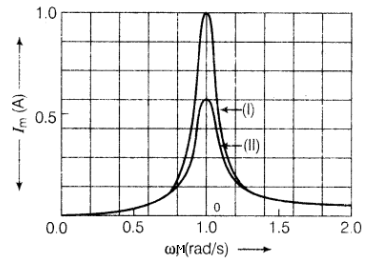# NEET Physics Alternating Current Questions SolvedBOARD

capacitor,  resistor and  inductor are connected in series with an AC source.

(i) What should be the frequency of the source such that current drawn in the circuit is maximum?

What is this frequency called ?

(ii) If the peak value of e.m.f. of the source is  find the maximum current.

(iii) Draw a graph showing variation of amplitude of circuit current with changing frequency of applied

voltage in a series LRC circuit for two different values of resistance ${\mathrm{R}}_{1}$ and ${\mathrm{R}}_{2}$ $\left({\mathrm{R}}_{1}>{\mathrm{R}}_{2}\right).$

(iv) Define the term 'Sharpness of Resonance'. Under what condition, does a circuit become more

selective ?                                                                                                   (5 marks)

(i)                             (1 marks)

Resonant frequency

(ii)    ${\mathrm{I}}_{\mathrm{max}}=\frac{{\mathrm{V}}_{0}}{\mathrm{R}}=\frac{200}{100}\mathrm{A}=2\mathrm{A}$                                                                             (1 marks)

(iii)(1 marks)

(iv)   $\frac{{\mathrm{\omega }}_{0}}{2∆\mathrm{\omega }}$ is measure of sharpness of resonance, where ${\mathrm{\omega }}_{0}$ is the resonant frequency and $2∆\mathrm{\omega }$ is

the resonant frequency and $2∆\mathrm{\omega }$ is the bandwidth.                                           (1 marks)

Circuit is more selective if it has greater value of sharpness/The circuit should have smaller

band width $∆\mathrm{\omega }.$

Difficulty Level:

Crack NEET with Online Course - Free Trial (Offer Valid Till September 21, 2019)